# High School Math : Solving Rational Equations and Inequalities

## Example Questions

### Example Question #101 : Intermediate Single Variable Algebra

Solve the following rational expression: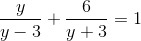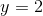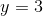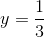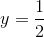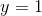Explanation:

Multiply the equation by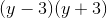: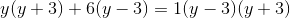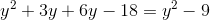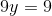### Example Question #1 : Solving Rational Equations And Inequalities

Solve the following rational equation: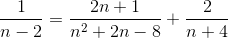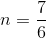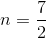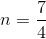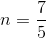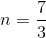Explanation:

Multiply the equation by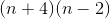:Simplify: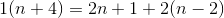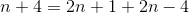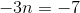### Example Question #3 : Solving Rational Equations And Inequalities

If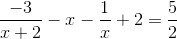, then what is the largest real value of x?Explanation:

We are asked to solve the equation.

Often, when solving equations involving rational expressions, it helps to elminate fractions by multiplying both sides of the equation by the denominators of each term intervolved.

In the context of this problem, we can first multiply both sides of the equation by x+2 to eliminate the denominator of the first term.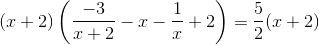Be sure to distribute the x+2 to each term on the left side of the equation.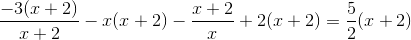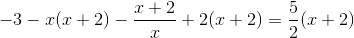Next, multiply both sides of the equation by x to elminate the term with an x in the denominator.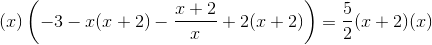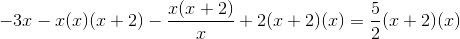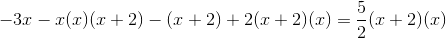Then, multiply both sides of the equation by 2.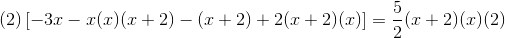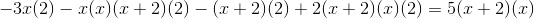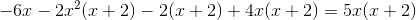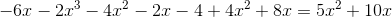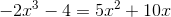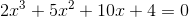In order to solve this polynomial equation, we can use the Rational Root Theorem. According to this theorem, if there is a rational root to a polynomial equation, then that root must be in the form p/q, where p is a factor of the constant, and q is the factor of the coefficient of the highest term.

In the context of this problem, p will be a factor of 4 (which is the constant), and q will be a factor of 2 (which is the coefficient of the highest term).

The factors of 4 are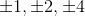. The factors of 2 are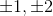.

This means that if there is a rational root for the equation, then the numerator must be either, and the denominator must be one of. In other words, the possible rational roots are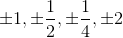, because these are all the possible ratios we can make with a numerator ofand a denominator of one of.

At this point, we have narrowed down the rational roots of the equationto the following eight choices:.

Notice, that if we were to let, then the polynomial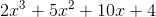would evaluate to 4. Also, if we were to let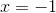, then the polynomial would evaluate to -3. Because the value of the polynomial switches from a postive value of 4 when x = 0 to a negative value of -3 when x = -1, there must be a root somewhere betweenandLet's see what happens when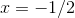, because -1/2 is between 0 and -1.

We will put -1/2 into the polynomial to see if it evaluates to zero.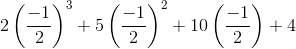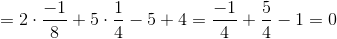Thusis indeed a root to the equation.

However, the question asks us to find the largest real root; this means that there could be other roots larger than. We will need to continue solving the equation to see what other roots are possible.

We can make use of the Factor Theorem of polynomials, which states that if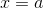is a root of a polynomial equation, where a is constant, then x-a must be a factor of the polynomial.

This means that for our polynomial,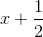is a factor. We can divide the polynomialbyusing long division.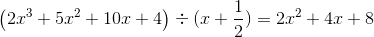This means that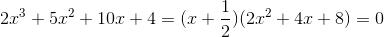In order to find the remaining roots of the equation, we now need to solve the quadratic equation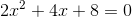.

First, divide both sides of the equation by two.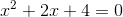Perhaps the most straightforward way to solve this is to use the quadratic formula: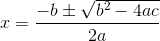When we evaluate this, we will be forced to take the square root of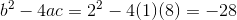. Because this will result in an imaginary number,has no real solutions.

Thus, returning to our original polynomial equation, it turns out that -1/2 is indeed the only root of the equation.

The question asks us to find the largest real value of x that solves the equation. Because -1/2 is the only real value that solves the equation, the answer must be -1/2.

### All High School Math Resources